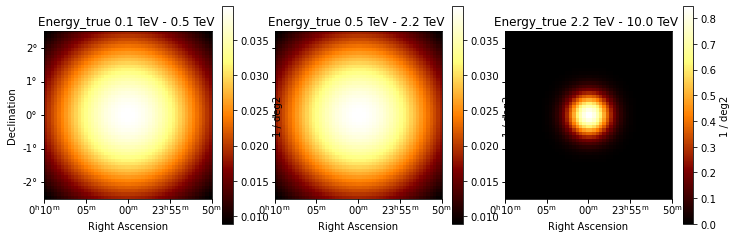This is a fixed-text formatted version of a Jupyter notebook

# Gammapy Models¶

This is an introduction and overview on how to work with models in Gammapy.

The sub-package gammapy.modeling contains all the functionality related to modeling and fitting data. This includes spectral, spatial and temporal model classes, as well as the fit and parameter API. We will cover the follwing topics in order:

The models follow a naming scheme which contains the category as a suffix to the class name. An overview of all the available models can be found in the model gallery.

Note that there is a separate tutorial modeling that explains about gammapy.modeling, the Gammapy modeling and fitting framework. You have to read that to learn how to work with models in order to analyse data.

# Setup¶

:

%matplotlib inline
import numpy as np
import matplotlib.pyplot as plt

:

from astropy import units as u
from gammapy.maps import Map, WcsGeom, MapAxis


# Spectral Models¶

All models are imported from the gammapy.modeling.models namespace. Let’s start with a PowerLawSpectralModel:

:

from gammapy.modeling.models import PowerLawSpectralModel

:

pwl = PowerLawSpectralModel()
print(pwl)

PowerLawSpectralModel

name     value         unit      min max frozen   error
--------- ---------- -------------- --- --- ------ ---------
index 2.0000e+00                nan nan  False 0.000e+00
amplitude 1.0000e-12 cm-2 s-1 TeV-1 nan nan  False 0.000e+00
reference 1.0000e+00            TeV nan nan   True 0.000e+00


To get a list of all available spectral models you can import and print the spectral model registry or take a look at the model gallery:

:

from gammapy.modeling.models import SPECTRAL_MODEL_REGISTRY

print(SPECTRAL_MODEL_REGISTRY)

Registry
--------

ConstantSpectralModel                  : ['ConstantSpectralModel', 'const']
CompoundSpectralModel                  : ['CompoundSpectralModel', 'compound']
PowerLawSpectralModel                  : ['PowerLawSpectralModel', 'pl']
PowerLaw2SpectralModel                 : ['PowerLaw2SpectralModel', 'pl-2']
BrokenPowerLawSpectralModel            : ['BrokenPowerLawSpectralModel', 'bpl']
SmoothBrokenPowerLawSpectralModel      : ['SmoothBrokenPowerLawSpectralModel', 'sbpl']
PiecewiseNormSpectralModel             : ['PiecewiseNormSpectralModel', 'piecewise-norm']
ExpCutoffPowerLawSpectralModel         : ['ExpCutoffPowerLawSpectralModel', 'ecpl']
ExpCutoffPowerLaw3FGLSpectralModel     : ['ExpCutoffPowerLaw3FGLSpectralModel', 'ecpl-3fgl']
SuperExpCutoffPowerLaw3FGLSpectralModel: ['SuperExpCutoffPowerLaw3FGLSpectralModel', 'secpl-3fgl']
SuperExpCutoffPowerLaw4FGLSpectralModel: ['SuperExpCutoffPowerLaw4FGLSpectralModel', 'secpl-4fgl']
LogParabolaSpectralModel               : ['LogParabolaSpectralModel', 'lp']
TemplateSpectralModel                  : ['TemplateSpectralModel', 'template']
GaussianSpectralModel                  : ['GaussianSpectralModel', 'gauss']
EBLAbsorptionNormSpectralModel         : ['EBLAbsorptionNormSpectralModel', 'ebl-norm']
NaimaSpectralModel                     : ['NaimaSpectralModel', 'naima']
ScaleSpectralModel                     : ['ScaleSpectralModel', 'scale']
PowerLawNormSpectralModel              : ['PowerLawNormSpectralModel', 'pl-norm']
LogParabolaNormSpectralModel           : ['LogParabolaNormSpectralModel', 'lp-norm']
ExpCutoffPowerLawNormSpectralModel     : ['ExpCutoffPowerLawNormSpectralModel', 'ecpl-norm']



Spectral models all come with default parameters. Different parameter values can be passed on creation of the model, either as a string defining the value and unit or as an astropy.units.Quantity object directly:

:

amplitude = 1e-12 * u.Unit("TeV-1 cm-2 s-1")
pwl = PowerLawSpectralModel(amplitude=amplitude, index=2.2)


For convenience a str specifying the value and unit can be passed as well:

:

pwl = PowerLawSpectralModel(amplitude="2.7e-12 TeV-1 cm-2 s-1", index=2.2)
print(pwl)

PowerLawSpectralModel

name     value         unit      min max frozen   error
--------- ---------- -------------- --- --- ------ ---------
index 2.2000e+00                nan nan  False 0.000e+00
amplitude 2.7000e-12 cm-2 s-1 TeV-1 nan nan  False 0.000e+00
reference 1.0000e+00            TeV nan nan   True 0.000e+00


The model can be evaluated at given energies by calling the model instance:

:

energy = [1, 3, 10, 30] * u.TeV
dnde = pwl(energy)
print(dnde)

[2.70000000e-12 2.40822469e-13 1.70358483e-14 1.51948705e-15] 1 / (cm2 s TeV)


The returned quantity is a differential photon flux.

For spectral models you can computed in addition the integrated and energy flux in a given energy range:

:

flux = pwl.integral(energy_min=1 * u.TeV, energy_max=10 * u.TeV)
print(flux)

eflux = pwl.energy_flux(energy_min=1 * u.TeV, energy_max=10 * u.TeV)
print(eflux)

2.108034597491956e-12 1 / (cm2 s)
4.982075849517389e-12 TeV / (cm2 s)


This also works for a list or an array of integration boundaries:

:

energy = [1, 3, 10, 30] * u.TeV
flux = pwl.integral(energy_min=energy[:-1], energy_max=energy[1:])
print(flux)

[1.64794383e-12 4.60090769e-13 1.03978226e-13] 1 / (cm2 s)


In some cases it can be useful to find use the inverse of a spectral model, to find the energy at which a given flux is reached:

:

dnde = 2.7e-12 * u.Unit("TeV-1 cm-2 s-1")
energy = pwl.inverse(dnde)
print(energy)

1.0 TeV


As a convenience you can also plot any spectral model in a given energy range:

:

pwl.plot(energy_range=[1, 100] * u.TeV)

:

<matplotlib.axes._subplots.AxesSubplot at 0x113c5a1d0>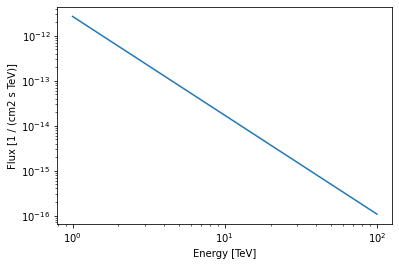# Spatial Models¶

Spatial models are imported from the same gammapy.modeling.models namespace, let’s start with a GaussianSpatialModel:

:

from gammapy.modeling.models import GaussianSpatialModel

:

gauss = GaussianSpatialModel(lon_0="0 deg", lat_0="0 deg", sigma="0.2 deg")
print(gauss)

GaussianSpatialModel

name   value    unit    min        max    frozen   error
----- ---------- ---- ---------- --------- ------ ---------
lon_0 0.0000e+00  deg        nan       nan  False 0.000e+00
lat_0 0.0000e+00  deg -9.000e+01 9.000e+01  False 0.000e+00
sigma 2.0000e-01  deg  0.000e+00       nan  False 0.000e+00
e 0.0000e+00       0.000e+00 1.000e+00   True 0.000e+00
phi 0.0000e+00  deg        nan       nan   True 0.000e+00


Again you can check the SPATIAL_MODELS registry to see which models are available or take a look at the model gallery.

:

from gammapy.modeling.models import SPATIAL_MODEL_REGISTRY

print(SPATIAL_MODEL_REGISTRY)

Registry
--------

ConstantSpatialModel           : ['ConstantSpatialModel', 'const']
TemplateSpatialModel           : ['TemplateSpatialModel', 'template']
DiskSpatialModel               : ['DiskSpatialModel', 'disk']
GaussianSpatialModel           : ['GaussianSpatialModel', 'gauss']
GeneralizedGaussianSpatialModel: ['GeneralizedGaussianSpatialModel', 'gauss-general']
PointSpatialModel              : ['PointSpatialModel', 'point']
ShellSpatialModel              : ['ShellSpatialModel', 'shell']



The default coordinate frame for all spatial models is "icrs", but the frame can be modified using the frame argument:

:

gauss = GaussianSpatialModel(
lon_0="0 deg", lat_0="0 deg", sigma="0.2 deg", frame="galactic"
)


You can specify any valid astropy.coordinates frame. The center position of the model can be retrieved as a astropy.coordinates.SkyCoord object using SpatialModel.position:

:

print(gauss.position)

<SkyCoord (Galactic): (l, b) in deg
(0., 0.)>


Spatial models can be evaluated again by calling the instance:

:

lon = [0, 0.1] * u.deg
lat = [0, 0.1] * u.deg

flux_per_omega = gauss(lon, lat)
print(flux_per_omega)

[13061.88470839 10172.60603928] 1 / sr


The returned quantity corresponds to a surface brightness. Spatial model can be also evaluated using gammapy.maps.Map and gammapy.maps.Geom objects:

:

m = Map.create(skydir=(0, 0), width=(1, 1), binsz=0.02, frame="galactic")
m.quantity = gauss.evaluate_geom(m.geom)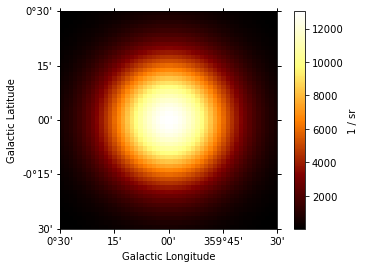Again for convenience the model can be plotted directly:

:

gauss.plot(add_cbar=True);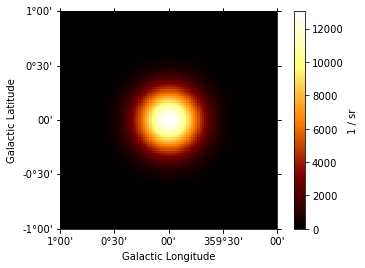All spatial models have an associated sky region to it e.g. to illustrate the extend of the model on a sky image. The returned object is an regions.SkyRegion object:

:

print(gauss.to_region())

Region: EllipseSkyRegion
center: <SkyCoord (Galactic): (l, b) in deg
(0., 0.)>
width: 0.4 deg
height: 0.4 deg
angle: 0.0 deg


Now we can plot the region on an sky image:

:

# create and plot the model
gauss_elongated = GaussianSpatialModel(
lon_0="0 deg", lat_0="0 deg", sigma="0.2 deg", e=0.7, phi="45 deg"
)

region = gauss_elongated.to_region()
region_pix = region.to_pixel(ax.wcs)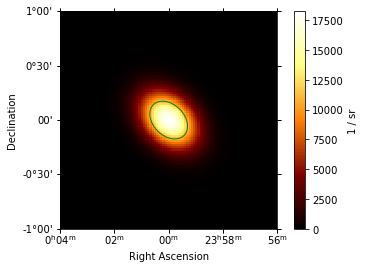The .to_region() method can also be useful to write e.g. ds9 region files using write_ds9 from the regions package:

:

from regions import write_ds9

regions = [gauss.to_region(), gauss_elongated.to_region()]

filename = "regions.reg"

:

!cat regions.reg

# Region file format: DS9 astropy/regions
galactic
ellipse(0.0000,0.0000,0.2000,0.2000,0.0000)
ellipse(96.3373,-60.1886,0.1428,0.2000,45.0000)


# SkyModel¶

The gammapy.modeling.models.SkyModel class combines a spectral and a spatial model. It can be created from existing spatial and spectral model components:

:

from gammapy.modeling.models import SkyModel

model = SkyModel(spectral_model=pwl, spatial_model=gauss, name="my-source")
print(model)

SkyModel

Name                      : my-source
Datasets names            : None
Spectral model type       : PowerLawSpectralModel
Spatial  model type       : GaussianSpatialModel
Temporal model type       :
Parameters:
index                   :   2.200
amplitude               :   2.70e-12  1 / (cm2 s TeV)
reference    (frozen)   :   1.000  TeV
lon_0                   :   0.000  deg
lat_0                   :   0.000  deg
sigma                   :   0.200  deg
e            (frozen)   :   0.000
phi          (frozen)   :   0.000  deg



It is good practice to specify a name for your sky model, so that you can access it later by name and have meaningful identifier you serilisation. If you don’t define a name, a unique random name is generated:

:

model_without_name = SkyModel(spectral_model=pwl, spatial_model=gauss)
print(model_without_name.name)

aNvy4ztk


The spectral and spatial component of the source model can be accessed using .spectral_model and .spatial_model:

:

model.spectral_model

:

<gammapy.modeling.models.spectral.PowerLawSpectralModel at 0x113c33f60>

:

model.spatial_model

:

<gammapy.modeling.models.spatial.GaussianSpatialModel at 0x113f32518>


And can be used as you have seen already seen above:

:

model.spectral_model.plot(energy_range=[1, 10] * u.TeV);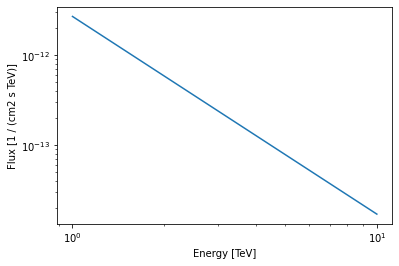In some cases (e.g. when doing a spectral analysis) there is only a spectral model associated with the source. So the spatial model is optional:

:

model_spectrum = SkyModel(spectral_model=pwl, name="source-spectrum")
print(model_spectrum)

SkyModel

Name                      : source-spectrum
Datasets names            : None
Spectral model type       : PowerLawSpectralModel
Spatial  model type       :
Temporal model type       :
Parameters:
index                   :   2.200
amplitude               :   2.70e-12  1 / (cm2 s TeV)
reference    (frozen)   :   1.000  TeV



Additionally the spatial model of gammapy.modeling.models.SkyModel can be used to represent source models based on templates, where the spatial and energy axes are correlated. It can be created e.g. from an existing FITS file:

:

from gammapy.modeling.models import TemplateSpatialModel
from gammapy.modeling.models import PowerLawNormSpectralModel

:

diffuse_cube = TemplateSpatialModel.read(
"$GAMMAPY_DATA/fermi-3fhl-gc/gll_iem_v06_gc.fits.gz", normalize=False ) diffuse = SkyModel(PowerLawNormSpectralModel(), diffuse_cube) print(diffuse)  SkyModel Name : etme4kaE Datasets names : None Spectral model type : PowerLawNormSpectralModel Spatial model type : TemplateSpatialModel Temporal model type : Parameters: norm : 1.000 tilt (frozen) : 0.000 reference (frozen) : 1.000 TeV  WARNING: FITSFixedWarning: 'datfix' made the change 'Set DATE-REF to '1858-11-17' from MJD-REF'. [astropy.wcs.wcs]  Note that if the spatial model is not normalized over the sky it has to be combined with a normalized spectral model, for example gammapy.modeling.models.PowerLawNormSpectralModel. This is the only case in gammapy.models.SkyModel where the unit is fully attached to the spatial model. # Model Lists and Serialisation¶ In a typical analysis scenario a model consists of mutiple model components, or a “catalog” or “source library”. To handle this list of multiple model components, Gammapy has a Models class: :  from gammapy.modeling.models import Models  :  models = Models([model, diffuse]) print(models)  Models Component 0: SkyModel Name : my-source Datasets names : None Spectral model type : PowerLawSpectralModel Spatial model type : GaussianSpatialModel Temporal model type : Parameters: index : 2.200 amplitude : 2.70e-12 1 / (cm2 s TeV) reference (frozen) : 1.000 TeV lon_0 : 0.000 deg lat_0 : 0.000 deg sigma : 0.200 deg e (frozen) : 0.000 phi (frozen) : 0.000 deg Component 1: SkyModel Name : etme4kaE Datasets names : None Spectral model type : PowerLawNormSpectralModel Spatial model type : TemplateSpatialModel Temporal model type : Parameters: norm : 1.000 tilt (frozen) : 0.000 reference (frozen) : 1.000 TeV  Individual model components in the list can be accessed by their name: :  print(models["my-source"])  SkyModel Name : my-source Datasets names : None Spectral model type : PowerLawSpectralModel Spatial model type : GaussianSpatialModel Temporal model type : Parameters: index : 2.200 amplitude : 2.70e-12 1 / (cm2 s TeV) reference (frozen) : 1.000 TeV lon_0 : 0.000 deg lat_0 : 0.000 deg sigma : 0.200 deg e (frozen) : 0.000 phi (frozen) : 0.000 deg  Note:To make the access by name unambiguous, models are required to have a unique name, otherwise an error will be thrown. To see which models are available you can use the .names attribute: :  print(models.names)  ['my-source', 'etme4kaE']  Note that a SkyModel object can be evaluated for a given longitude, latitude, and energy, but the Models object cannot. This Models container object will be assigned to Dataset or Datasets together with the data to be fitted as explained in other analysis tutorials (see for example the modeling notebook). The Models class also has in place .append() and .extend() methods: :  model_copy = model.copy(name="my-source-copy") models.append(model_copy)  This list of models can be also serialised to a custom YAML based format: :  models_yaml = models.to_yaml() print(models_yaml)  components: - name: my-source type: SkyModel spectral: type: PowerLawSpectralModel parameters: - name: index value: 2.2 - name: amplitude value: 2.7e-12 unit: cm-2 s-1 TeV-1 - name: reference value: 1.0 unit: TeV frozen: true spatial: type: GaussianSpatialModel frame: galactic parameters: - name: lon_0 value: 0.0 unit: deg - name: lat_0 value: 0.0 unit: deg - name: sigma value: 0.2 unit: deg - name: e value: 0.0 frozen: true - name: phi value: 0.0 unit: deg frozen: true - name: etme4kaE type: SkyModel spectral: type: PowerLawNormSpectralModel parameters: - name: norm value: 1.0 - name: tilt value: 0.0 frozen: true - name: reference value: 1.0 unit: TeV frozen: true spatial: type: TemplateSpatialModel frame: galactic parameters: [] filename: /Users/adonath/Desktop/gammapy-tutorials/datasets/fermi-3fhl-gc/gll_iem_v06_gc.fits.gz normalize: false unit: 1 / (cm2 MeV s sr) - name: my-source-copy type: SkyModel spectral: type: PowerLawSpectralModel parameters: - name: index value: 2.2 - name: amplitude value: 2.7e-12 unit: cm-2 s-1 TeV-1 - name: reference value: 1.0 unit: TeV frozen: true spatial: type: GaussianSpatialModel frame: galactic parameters: - name: lon_0 value: 0.0 unit: deg - name: lat_0 value: 0.0 unit: deg - name: sigma value: 0.2 unit: deg - name: e value: 0.0 frozen: true - name: phi value: 0.0 unit: deg frozen: true  The structure of the yaml files follows the structure of the python objects. The components listed correspond to the SkyModel and SkyDiffuseCube components of the Models. For each SkyModel we have informations about its name, type (corresponding to the tag attribute) and sub-mobels (i.e spectral model and eventually spatial model). Then the spatial and spectral models are defiend by their type and parameters. The parameters keys name/value/unit are mandatory, while the keys min/max/frozen are optionnals (so you can prepare shorter files). If you want to write this list of models to disk and read it back later you can use: :  models.write("models.yaml", overwrite=True)  :  models_read = Models.read("models.yaml")  WARNING: FITSFixedWarning: 'datfix' made the change 'Set DATE-REF to '1858-11-17' from MJD-REF'. [astropy.wcs.wcs]  Additionally the models can exported and imported togeter with the data using the Datasets.read() and Datasets.write() methods as shown in the analysis_mwl notebook. # Implementing a Custom Model¶ In order to add a user defined spectral model you have to create a SpectralModel subclass. This new model class should include: • a tag used for serialization (it can be the same as the class name) • an instantiation of each Parameter with their unit, default values and frozen status • the evaluate function where the mathematical expression for the model is defined. As an example we will use a PowerLawSpectralModel plus a Gaussian (with fixed width). First we define the new custom model class that we name MyCustomSpectralModel: :  from gammapy.modeling.models import SpectralModel, Parameter class MyCustomSpectralModel(SpectralModel): """My custom spectral model, parametrising a power law plus a Gaussian spectral line. Parameters ---------- amplitude : astropy.units.Quantity Amplitude of the spectra model. index : astropy.units.Quantity Spectral index of the model. reference : astropy.units.Quantity Reference energy of the power law. mean : astropy.units.Quantity Mean value of the Gaussian. width : astropy.units.Quantity Sigma width of the Gaussian line. """ tag = "MyCustomSpectralModel" amplitude = Parameter("amplitude", "1e-12 cm-2 s-1 TeV-1", min=0) index = Parameter("index", 2, min=0) reference = Parameter("reference", "1 TeV", frozen=True) mean = Parameter("mean", "1 TeV", min=0) width = Parameter("width", "0.1 TeV", min=0, frozen=True) @staticmethod def evaluate(energy, index, amplitude, reference, mean, width): pwl = PowerLawSpectralModel.evaluate( energy=energy, index=index, amplitude=amplitude, reference=reference, ) gauss = amplitude * np.exp(-((energy - mean) ** 2) / (2 * width ** 2)) return pwl + gauss  It is good practice to also implement a docstring for the model, defining the parameters and also definig a tag, which specifies the name of the model for serialisation. Also note that gammapy assumes that all SpectralModel evaluate functions return a flux in unit of "cm-2 s-1 TeV-1" (or equivalent dimensions). This model can now be used as any other spectral model in Gammapy: :  my_custom_model = MyCustomSpectralModel(mean="3 TeV") print(my_custom_model)  MyCustomSpectralModel name value unit min max frozen error --------- ---------- -------------- --------- --- ------ --------- amplitude 1.0000e-12 cm-2 s-1 TeV-1 0.000e+00 nan False 0.000e+00 index 2.0000e+00 0.000e+00 nan False 0.000e+00 reference 1.0000e+00 TeV nan nan True 0.000e+00 mean 3.0000e+00 TeV 0.000e+00 nan False 0.000e+00 width 1.0000e-01 TeV 0.000e+00 nan True 0.000e+00  :  my_custom_model.integral(1 * u.TeV, 10 * u.TeV)  :  $$1.1442739 \times 10^{-12} \; \mathrm{\frac{1}{s\,cm^{2}}}$$ :  my_custom_model.plot(energy_range=[1, 10] * u.TeV)  :  <matplotlib.axes._subplots.AxesSubplot at 0x114462978>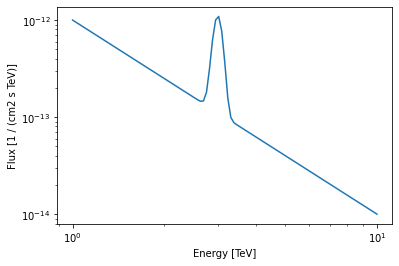As a next step we can also register the custom model in the SPECTRAL_MODELS registry, so that it becomes available for serilisation: :  SPECTRAL_MODEL_REGISTRY.append(MyCustomSpectralModel)  :  model = SkyModel(spectral_model=my_custom_model, name="my-source") models = Models([model]) models.write("my-custom-models.yaml", overwrite=True)  :  !cat my-custom-models.yaml  components: - name: my-source type: SkyModel spectral: type: MyCustomSpectralModel parameters: - name: amplitude value: 1.0e-12 unit: cm-2 s-1 TeV-1 - name: index value: 2.0 - name: reference value: 1.0 unit: TeV frozen: true - name: mean value: 3.0 unit: TeV - name: width value: 0.1 unit: TeV frozen: true covariance: my-custom-models_covariance.dat  Similarly you can also create custom spatial models and add them to the SPATIAL_MODELS registry. In that case gammapy assumes that the evaluate function return a normalized quantity in “sr-1” such as the model integral over the whole sky is one. # Models with Energy dependent morphology¶ A common science case in the study of extended sources is to probe for energy dependent morphology, in Supernova Remnants or Pulsar Wind Nebulae. Traditionally, this has been done by splitting the data into energy bands and doing individual fits of the morphology in these energy bands. SkyModel offers a natural framework to simultaneously model the energy and morphology, e.g. spatial extent described by a parametric model expression with energy dependent parameters. The models shipped within gammapy use a “factorised” representation of the source model, where the spatial ($$l,b$$), energy ($$E$$) and time ($$t$$) dependence are independent model components and not correlated: $f(l, b, E, t) = F(l, b) \cdot G(E) \cdot H(t)$ To use full 3D models, ie$f(l, b, E) = F(l, b, E) \cdot G(E) \$, you have to implement your own custom SpatialModel. Note that it is still necessary to multiply by a SpectralModel, $$G(E)$$ to be dimensionally consistent.

In this example, we create Gaussian Spatial Model with the extension varying with energy. For simplicity, we assume a linear dependence on energy and parameterize this by specifing the extension at 2 energies. You can add more complex dependences, probably motivated by physical models.

:

from gammapy.modeling.models import SpatialModel
from astropy.coordinates.angle_utilities import angular_separation

class MyCustomGaussianModel(SpatialModel):
"""My custom Energy Dependent Gaussian model.

Parameters
----------
lon_0, lat_0 : ~astropy.coordinates.Angle
Center position
sigma_1TeV : ~astropy.coordinates.Angle
Width of the Gaussian at 1 TeV
sigma_10TeV : ~astropy.coordinates.Angle
Width of the Gaussian at 10 TeV

"""

tag = "MyCustomGaussianModel"
lon_0 = Parameter("lon_0", "0 deg")
lat_0 = Parameter("lat_0", "0 deg", min=-90, max=90)

sigma_1TeV = Parameter("sigma_1TeV", "2.0 deg", min=0)
sigma_10TeV = Parameter("sigma_10TeV", "0.2 deg", min=0)

@staticmethod
def get_sigma(energy, sigma_1TeV, sigma_10TeV):
"""Get the sigma for a particular energy"""
sigmas = u.Quantity([sigma_1TeV, sigma_10TeV])
energy_nodes = [1, 10] * u.TeV

log_s = np.log(sigmas.to("deg").value)
log_en = np.log(energy_nodes.to("TeV").value)
log_e = np.log(energy.to("TeV").value)
return np.exp(np.interp(log_e, log_en, log_s)) * u.deg

def evaluate(
self, lon, lat, energy, lon_0, lat_0, sigma_1TeV, sigma_10TeV
):
"""Evaluate custom Gaussian model"""

sigma = self.get_sigma(energy, sigma_1TeV, sigma_10TeV)
sep = angular_separation(lon, lat, lon_0, lat_0)

exponent = -0.5 * (sep / sigma) ** 2
norm = 1 / (2 * np.pi * sigma ** 2)
return norm * np.exp(exponent)

@property
"""Evaluation radius (~astropy.coordinates.Angle)."""
return (
5 * np.max([self.sigma_1TeV.value, self.sigma_10TeV.value]) * u.deg
)


Serialisation of this model can be achieved as explained in the previous section. You can now use it as stadard SpatialModel in your analysis. Note that this is still a SpatialModel, and not a SkyModel, so it needs to be multiplied by a SpectralModel as before.

:

spatial_model = MyCustomGaussianModel()
spectral_model = PowerLawSpectralModel()
sky_model = SkyModel(
spatial_model=spatial_model, spectral_model=spectral_model
)


To visualise it, we evaluate it on a 3D geom.

:

energy_axis = MapAxis.from_energy_bounds(
energy_min=0.1 * u.TeV, energy_max=10.0 * u.TeV, nbin=3, name="energy_true"
)
geom = WcsGeom.create(
skydir=(0, 0), width=5.0 * u.deg, binsz=0.1, axes=[energy_axis]
)